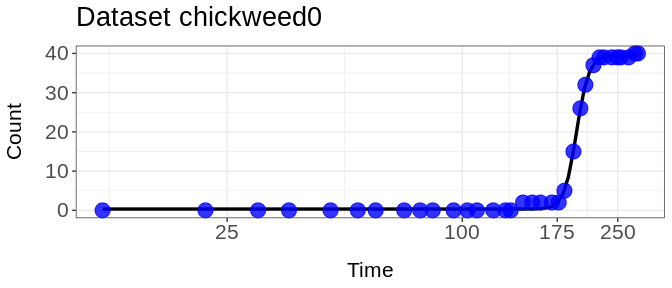R package dr4pl usage examples

2019-10-07

##Installing packages

Our R package dr4pl is now available on CRAN! You can download it by the command install.packages(“dr4pl”). Note that the argument repos can be omitted. Let us now load R packages that we need to use in our examples.

library(drc)
##
## Please cite R and 'drc' if used for a publication,
## for references type 'citation()' and 'citation('drc')'.
##
## Attaching package: 'drc'
## The following objects are masked from 'package:stats':
##
##     gaussian, getInitial
library(dr4pl)
library(ggplot2)

dr4pl is an abbreviation for Dose-Response data analysis using 4 Parameter Logistic (4PL) model. 4PL models are typically used models in pharmacological and toxicological experiments.

##Errors drawn by the R package ‘drc’

The most well-known R package for dose-response modelling is drc. However, that R package has drawn errors in many data sets generated by Dittmer Lab. Let’s first consider the first error case drc_error_1.

##   Dose Response
## 1    1        0
## 2    1       26
## 3    1       12
## 4    1        0
## 5    1       48
## 6    1       21
ggplot(drc_error_1, aes(x = Dose, y = Response)) +  # Data name, variable names
geom_point() +  # Put data points on the scatter plot
scale_x_log10() +  # Change the x-axis scale to log 10 scale
ggtitle("Error Case 1")  # Set the title
## Warning: Transformation introduced infinite values in continuous x-axis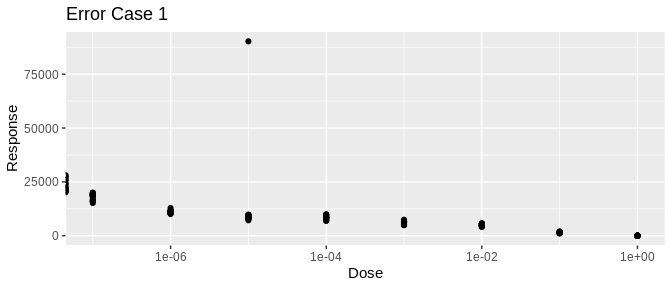As you can see, this data set contains an extreme outlier at the dosage level 1e-05. These outliers are often generated by measurement errors or experimental failure. If you were to fit a 4PL model with drc, you would recieve the following.

tryCatch({
drm(Response~Dose, data = drc_error_1, fct = LL.4())
},
warning = function(war) {
# warning handler picks up where error was generated
print(paste(sep = " ", war))
},
error = function(err) {
# error handler picks up where error was generated
print(paste(sep = " ", err))
})
##  "simpleWarning in log(dose/parmMat[, 4]): NaNs produced\n"

Note that the error message just indicates convergence failure without explanation about the reasons. Instead of giving up, you can try to use dr4pl.

dr4pl.error.1 <- dr4pl(Response~Dose, data = drc_error_1)

plot(dr4pl.error.1, text.title = "Error plot #1")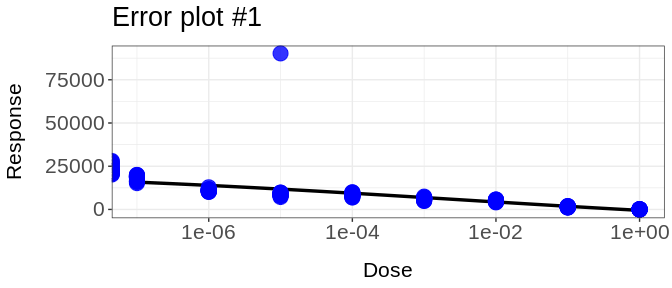The fitted curve does not look bad. Unlike drc, convergence failure does not draw an error but presents FALSE value in an indicator variable.

dr4pl.error.1$convergence # FALSE indicates convergence failure ##  FALSE Once convergence failure happens, dr4pl returns a child object which is obtained by robust estimation. dr4pl.robust.1 <- dr4pl.error.1$dr4pl.robust
class(dr4pl.error.1)
##  "dr4pl"
class(dr4pl.robust.1)
##  "dr4pl"
dr4pl.robust.1$convergence ##  TRUE The parent object dr4pl.error.1 and the child object dr4pl.robust.1 have the same class dr4pl. Note that the child object dr4pl.robust.1 indicates that convergence failure did not happen when robust estimation is applied. In the following diagnosis plot, outliers are denoted by red triangles. plot(dr4pl.robust.1, indices.outlier = dr4pl.robust.1$idx.outlier)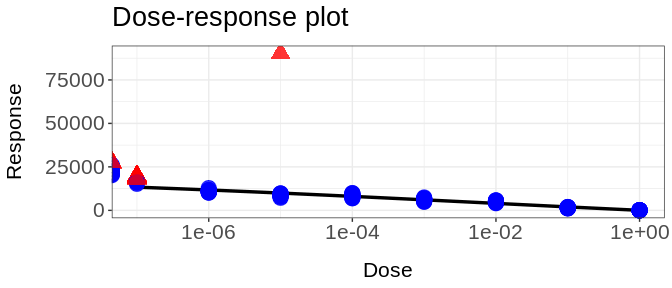Let’s next consider the second error case drc_error_2.

ggplot(drc_error_2, aes(x = Dose, y = Response)) +
geom_point() +
scale_x_log10() +
ggtitle("Error Case 2")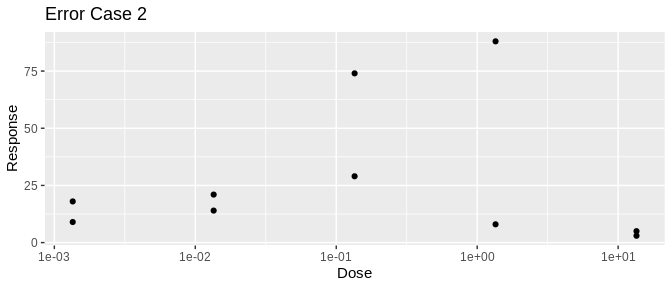The most important problem with Error Case 2 is that whether its trend is decreasing or increasing is ambiguous. dr4pl’s default parameter trend automizes this and will find the best fit based on optimization. Let us see how this example plays out with the data set drc_error_2.

dr4pl.error.2 <- dr4pl(Response~Dose, data = drc_error_2, method.init = "logistic")
plot(dr4pl.error.2, text.title = "Trend is default")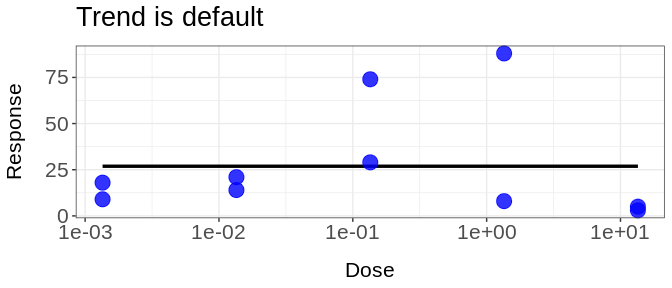The parameter trend helps force the fit to either increasing or decreasing in the event that you are confident that your response follows that trend. We may choose to force a decreasing curve, with other apporpriate parameters.

dr4pl.error.2 <- dr4pl(Response~Dose, data = drc_error_2, trend = "decreasing", method.init = "logistic", method.robust = "absolute")
plot(dr4pl.error.2, text.title = "Trend forced to decrease")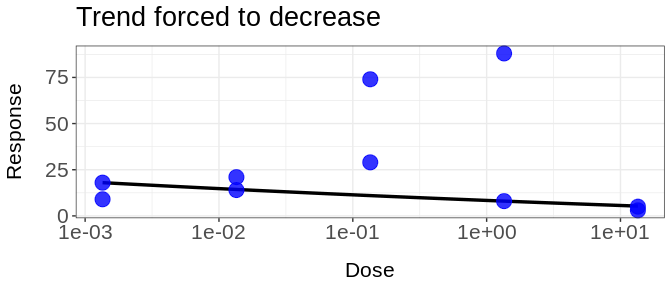Note that the fit now is decreasing. Let’s consider the data set drc_error_4.

ggplot(drc_error_4, aes(x = Dose, y = Response)) +
geom_point() +
scale_x_log10() +
ggtitle("drc_error_4")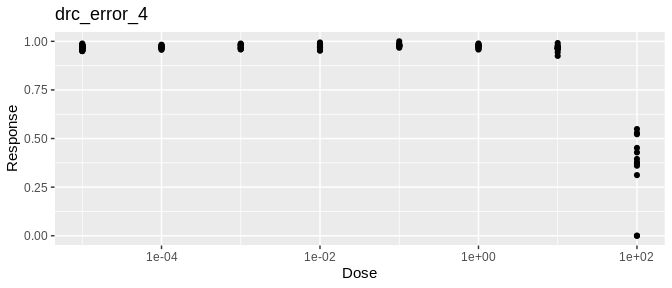This data set has two outliers in the largest dosage level. This data set also exemplifies the support problem as well. A support problem indicates that a data set does not have enough data points on the right side of the IC50 parameter. This can result in difficulty in sometimes obtaining estimates of the lower asymptote and slope and sometimes even convergence failure. Let’s try to apply drc to this data set.

tryCatch({
drm(Response~Dose, data = drc_error_4, fct = LL.4())
},
warning = function(war) {
# warning handler picks up where error was generated
print(paste(sep = " ", war))
},
error = function(err) {
# error handler picks up where error was generated
print(paste(sep = " ", err))
})
##  "simpleWarning in log(dose/parmMat[, 4]): NaNs produced\n"

As expected, drc is not able to fit a 4PL model to drc_error_4. Now let’s use dr4pl.

dr4pl.error.4 <- dr4pl(Response~Dose, data = drc_error_4, method.init = "logistic")
dr4pl.error.4$convergence ##  TRUE plot(dr4pl.error.4, text.title = "Error plot #4")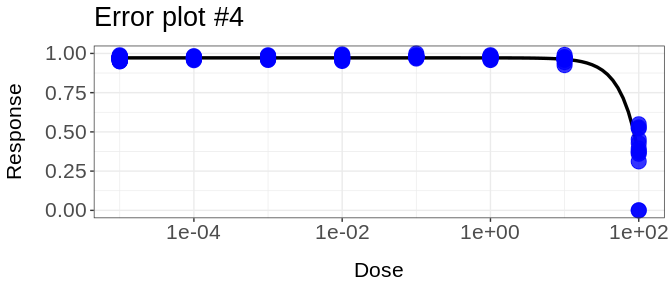The package drc draws errors with each one of these cases. However dr4pl is able generate a curve despite outliers and a support problem in each error case. In each case we were able to modify the title and axis names with text.title, text.x, or text.y. We are also able to bring attention to outlier points by passing a vector of the indices to the indices.outlier argument. ##Robust Estimation dr4pl provides several methods of robust estimation. Consider you want to plot the dr4pl data set sample_data_5. Let’s try and apply the default parameters. dr4pl.Mead.5 <- dr4pl(Response~Dose, data = sample_data_5) plot(dr4pl.Mead.5, text.title = "Fit with Mead's method")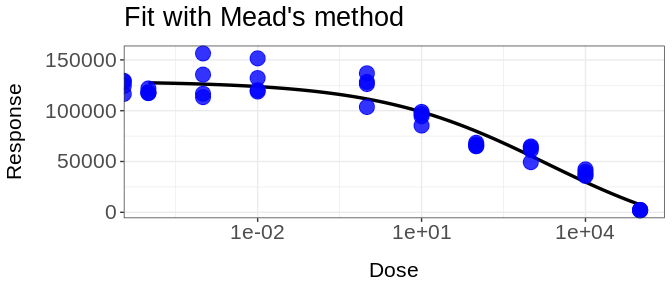After producing a plot with the default parameters, you may be confident in producing a better fit with other parameters. This is where the arguments method.init and method.robust come into play, which you may have noticed that these arguments where used in the error case examples. The default paramter to method.init is Mead. This uses Mead’s method when approximating the theta parameters. The alternative to using Mead’s method is logistic, which uses a logistic method to obtain initial parameter estimates. Let’s see how our plot will change when we use the logistic method. dr4pl.logistic.5 <- dr4pl(Response~Dose, data = sample_data_5, method.init = "logistic") plot(dr4pl.logistic.5, text.title = "Fit with the logistic method")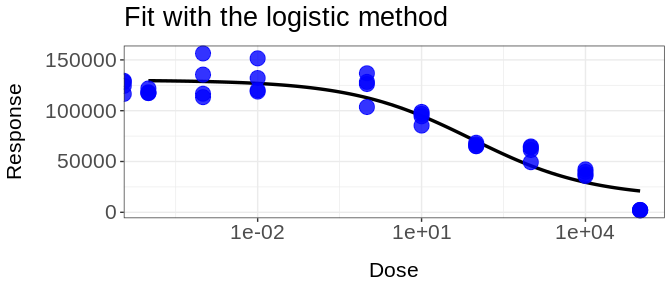Mead’s method seems to generate tighter fit with this data set. This is due to a characteristic of Mead’s method that it focuses more on the slope and lower asymptote parameters than the logistic method. Now let’s talk about method.robust. method.robust allows the user to choose the loss function used during optimization. The default loss function used is the squared loss function. The user has three other loss functions to select from: absolute, Huber, and Tukey. Lets see how this plot will change when using absolute versus Tukey. dr4pl.absolute.5 <- dr4pl(Response~Dose, data = sample_data_5, method.robust = "absolute") plot(dr4pl.absolute.5, text.title = "Fit with the absolute loss")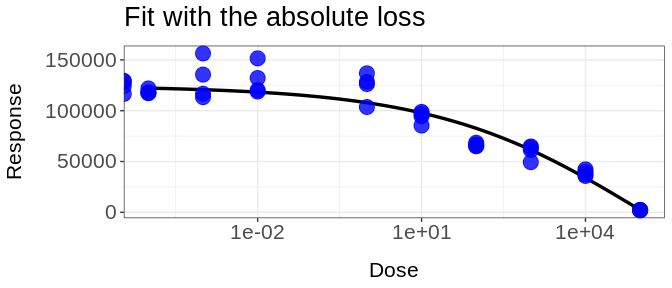dr4pl.Tukey.5 <- dr4pl(Response~Dose, data = sample_data_5, method.robust = "Tukey") plot(dr4pl.Tukey.5, text.title = "Fit with Tukey's biweight loss")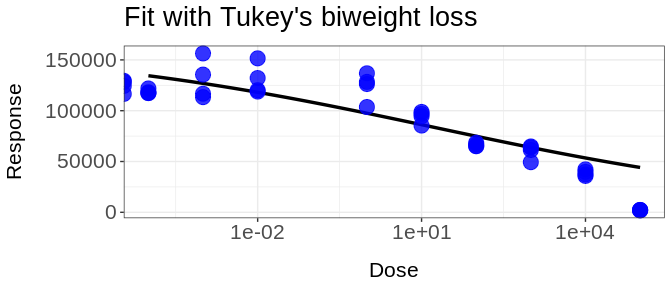When selecting Tukey for the argument method.robust, the generated fit is less weighted by the largest dosage level which is an outlier response of zero. When selecting absolute for the argument method.robust, the generated fit is more weignted by the largest dosage level. It may take several attempts to find the best parameters for each data set. ##Other functionalities of dr4pl Let’s look at other uses for this code. Consider the following data set sample_data_3. dr4pl.3 <- dr4pl(Response~Dose, data = sample_data_3) plot(dr4pl.3, text.title = "Sample data #3")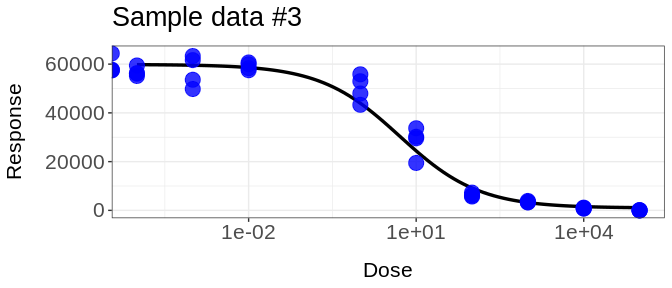Once you produce a curve you feel is representative of your data, you may get the parameters of the curve by using the summary function on the dr4pl variable. summary.dr4pl.3 <- summary(dr4pl.3) summary.dr4pl.3$coefficients
##                 Estimate       StdErr         2.5 %        97.5 %
## UpperLimit 59858.0700194 1.328219e-01 58678.1496415 61037.9903974
## IC50           5.0794918 1.531608e-05     3.7133017     6.9483278
## Slope         -0.6117371 1.440712e-05    -0.7397224    -0.4837518
## LowerLimit   980.8205686 1.354448e-01  -222.4002527  2184.0413899

It is possible that you are interested in more than just the IC50 variable. Use the IC() function to produce the respective dose values.

values <- IC(dr4pl.3, c(10, 30, 50, 70, 90))
values
## InhibPercent:10 InhibPercent:30 InhibPercent:50 InhibPercent:70
##     184.3784198      20.2930770       5.0794918       1.2714305
## InhibPercent:90
##       0.1399363

Finally, you can easily edit your plot further with basic ggplot2 additional controls. Let’s see how dr4pl handles the data set chekcweed0 in drc. As can be seen in the figure, a user can set the x-axis and y-axis labels and also the x-axis tick labels.

dr4pl.chickweed <- dr4pl(count~time, data = chickweed0, trend = "increasing")
plot(dr4pl.chickweed, text.x = "Time", text.y = "Count", text.title = "Dataset chickweed0", breaks.x = c(25, 100, 175, 250))